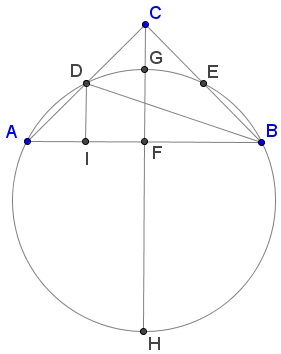# Golden Ratio In Right Isosceles Triangle

The following has been posted by Tran Quang Hung at the CutTheKnotMath facebook page.

Let $ABC\;$ be an Right Isosceles triangle, $D\;$ and $E\;$ the midpoints of the sides; $F\;$ be the foot of the latitude from $C;\;$ $G\;$ the intersection of $CF\;$ and circle $(ABED).$Then $G\;$ divides $CF\;$ in the Golden Ratio: $\displaystyle\frac{FG}{CG}=\varphi.$

Assume $AB=4\;$ and expand the diagram as shown:Then $AI=DI=1;\;$ $AD=\sqrt{2};\;$ $BI=3;\;$ $BD=\sqrt{10};\;$ area $S=[\Delta ABD]=2,\;$ and from $abc=4RS,$ the circumradius $R\;$ (i.e., the radius of $(ABED))$ is $\displaystyle R=\frac{\sqrt{2}\cdot 4\cdot\sqrt{10}}{4\cdot 2}=\sqrt{5}.$

By the Power of a Point Theorem, for $C,\;$ $AC\cdot CD=CG\cdot CH.\;$ With $x=CG,\;$ this becomes $4=x(x+2\sqrt{5}),\;$ so that $x=-\sqrt{5}+3.\;$ Since $CF=2,\;$ $\displaystyle\frac{FG}{CG}=\frac{\sqrt{5}-1}{3-\sqrt{5}}=\varphi.$

Grégoire Nicollier has observed that a shorter proof follows from the fact that the center of the circle lies $1\;$ unit below $F\;$ because this point is on equal distance $\sqrt{5}\;$ from $A,\;$ $B,\;$ $E,\;$ and $D.\;$ Then it is immediate that $FG=\sqrt(5)-1\;$ and $GC = 2-FG.$### Golden Ratio